# Phase Estimation Algorithm

## Rotation Matrix

We use a rotation matrix $U\ =\ \begin{pmatrix} c & s \\ -s & c \\ \end{pmatrix}$ with $$c=\cos(\alpha)$$, $$s=\sin(\alpha)$$ and a real-valued angle $$\alpha$$ as an example. $$U$$ has eigenvalues $\lambda_\pm\ =\ c\pm \mathrm{i} s\ =\ e^{\pm i \alpha}\,.$ Thus, $$\phi=\alpha/(2\pi)$$. The corresponding eigenvectors are of the form $u_\pm\ =\ \begin{pmatrix} 1 \\ \pm\mathrm{i}\\ \end{pmatrix}\,.$

## Phase Estimation

We use

t=6

in the second register which allows us with probability $$1-\epsilon$$ to get the correct phase up to $$t-\left\lceil \log\left(2+\frac{1}{2\epsilon}\right)\right\rceil$$ digits. Let us choose

epsilon <- 1/4
digits <- t-ceiling(log(2+1/(2*epsilon))/log(2))
digits
 4

and therefore expect an error of less than

2^(-digits)
 0.0625

We start with qubit 1 in state $$u_+$$

x <- S(1) * (H(1) * qstate(t+1, basis=""))

and we define the gate corresponding to $$U$$

alpha <- pi*3/7
s <- sin(alpha)
c <- cos(alpha)
## note that R fills the matrix columns first
M <- array(as.complex(c(c, -s, s, c)), dim=c(2,2))
Uf <- sqgate(bit=1, M=M, type=paste0("Uf"))

Now we apply the Hadamard gate to qubits 2,,t+1

for(i in c(2:(t+1))) {
x <- H(i) * x
}

and the controlled $$U_f$$

for(i in c(2:(t+1))) {
x <- cqgate(bits=c(i, 1),
gate=sqgate(bit=1,
M=M, type=paste0("Uf", 2^(i-2)))) * x
M <- M %*% M
}
plot(x)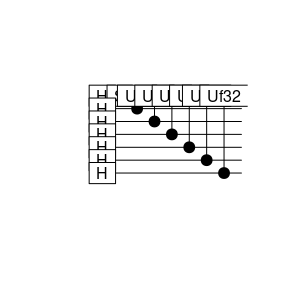Next we apply the inverse Fourier transform

x <- qft(x, inverse=TRUE, bits=c(2:(t+1)))
plot(x)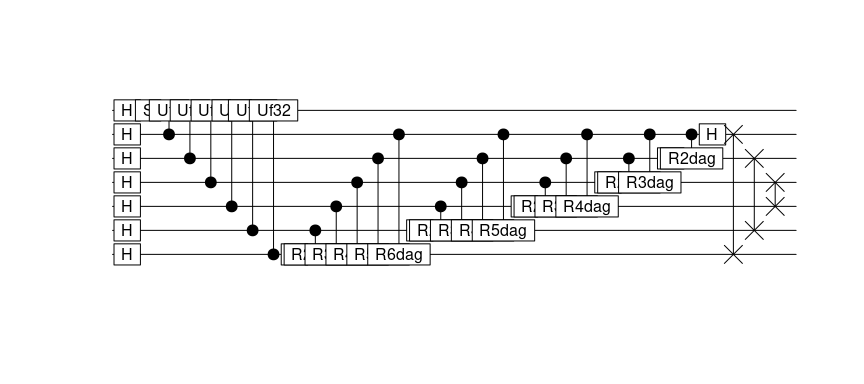$$x$$ is now the state $$|\tilde\varphi\rangle|u\rangle$$. $$|\tilde\varphi\rangle$$ is not necessarily a pure state. The next step is a projective measurement of $$|\tilde\varphi\rangle$$

xtmp <- measure(x)
cbits <- genStateNumber(which(xtmp$value==1)-1, t+1) phi <- sum(cbits[1:t]/2^(1:t)) cbits[1:t]  0 0 1 1 1 0 phi  0.21875 Note that we can measure the complete state, because $$|u\rangle$$ is not entangled to the rest. We find that usually phi-alpha/(2*pi)  0.004464286 is indeed smaller than the maximal deviation $$2^{-\mathrm{digits}}=$$ 0.0625 we expect. The distribution of probabilities over the states in $$|\tilde\varphi\rangle$$ is given as follows (factor 2 from dropping $$|u\rangle$$) plot(2*abs(x@coefs[seq(1,128,2)])^2, type="l", ylab="p", xlab="state index")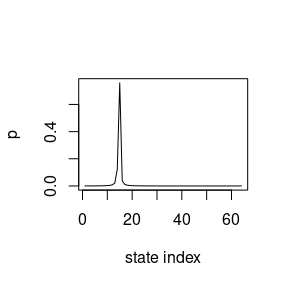## Starting from a random state The algorithm also works in case the specific eigenvector cannot be prepared. Starting with a random initial state $$|\psi\rangle = \sum_u c_u |u\rangle$$, we may apply the very same algorithm and we will find the approximation to the phase $$\varphi_u$$ with probability $$|c_u|^2(1-\epsilon)$$. We prepare the second register in the state $\begin{pmatrix} 1\\ 1\\ \end{pmatrix}\ =\ (1-i) u_+ + (1+i) u_-\,.$ x <- (H(1) * qstate(t+1, basis="")) This implies that we will find both $$\varphi_u$$ with equal probability. for(i in c(2:(t+1))) { x <- H(i) * x } M <- array(as.complex(c(c, -s, s, c)), dim=c(2,2)) for(i in c(2:(t+1))) { x <- cqgate(bits=c(i, 1), gate=sqgate(bit=1, M=M, type=paste0("Uf", 2^(i-2)))) * x M <- M %*% M } x <- qft(x, inverse=TRUE, bits=c(2:(t+1))) measurephi <- function(x, t) { xtmp <- measure(x) cbits <- genStateNumber(which(xtmp$value==1)-1, t+1)
phi <- sum(cbits[1:t]/2^(1:t))
return(invisible(phi))
}
phi <- measurephi(x, t=t)
2*pi*phi
 1.374447
phi-c(+alpha, 2*pi-alpha)/2/pi
  0.004464286 -0.566964286

We can draw the probability distribution again and observe the two peaks corresponding to the two eigenvalues

plot(abs(x@coefs)^2, type="l",
ylab="p", xlab="state index")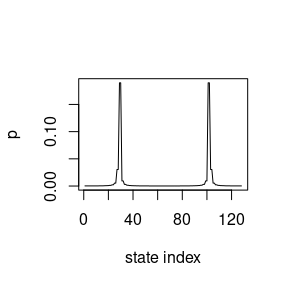Let’s measure 1000 times, which is easily possible in our simulator

phi <- c()
for(i in c(1:N)) {
phi[i] <- measurephi(x, t)
}
hist(phi, breaks=2^t, xlim=c(0,1))
abline(v=c(alpha/2/pi, 1-alpha/2/pi), lwd=2, col="red")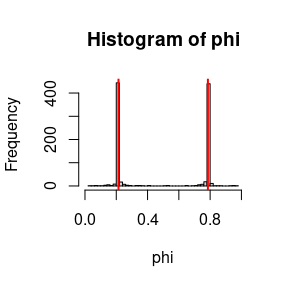The red vertical lines indicate the true values.